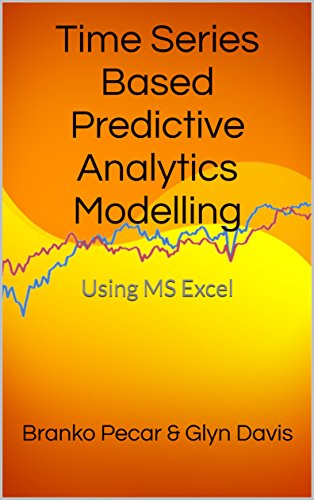Table of Content - Time Series Based Predictive Analytics Modelling in Excel
Welcome to "Statistics for Business Students" Textbook
Introduction
1. Predictive analytics in a broader context
1.1 Data mining and Data analytics
1.2 Business Intelligence and Decision Support Systems
1.3 Predictive analytics subset
1.4 Relationship between forecasting, planning and decision-making
2. Predictive analytics modelling rationale and basic ideas
2.1 Time series based forecasting models
2.2 Forecasting horizon
2.3 Data inspection and preparation
3. The role of error measurement
3.1 Error measurement
3.2 Types of errors
3.3 Other error metrics
4. Understanding basic techniques
4.1 Averaging
4.2 Differencing
4.3 Transformations
4.4 Smoothing
5. Associations between and within the time series
5.1 Correlation
5.2 Autocorrelation
5.3 Interpreting autocorrelations
5.4 Corrections to the autocorrelations standard error
5.5 Partial Autocorrelation
5.6 Using Excel functions to calculate partial autocorrelations
5.7 Standard errors for the partial autocorrelations
5.8 Autocorrelations evaluation methods
6. Long-term models - Fitting underlying trend and regression
6.1 Visualisation
6.2 Time series classification
6.3 Curve classification
6.4 Recognising curves
6.5 Fitting curves and extrapolating
6.6 Simple Regression
6.6.1 Variations around the regression line
6.6.2 Estimating parameters
6.6.4 Basic confidence interval
6.6.5 Changing confidence interval
6.6.6 The alternative confidence interval calculation
6.7 Inspecting Errors in Regression Analysis
6.7.1 Durbin-Watson test
6.7.2 Homoscedasticity
7. Short-term models
7.1 Simple averaging techniques
7.2 Exponential Smoothing
7.2.1 The Standard Errors
8. Medium-term models
8.1 Double moving averages (DMA)
8.2 Double exponential smoothing (DES)
8.3 Triple exponential smoothing (TES)
8.4 Holt’s Double exponential smoothing method
8.5 Damped trend exponential smoothing methods
9. Seasonal models
9.1. Classical decomposition method
9.2 Seasonal adjustments and exponential smoothing
9.3 Simple seasonal exponential smoothing
9.4 Holt-Winters seasonal method

10. State space models
10.1 Defining a state space model
10.2 Excel implementation of the ETS(A,A,A) models
10.3 Manual state space modelling in Excel
10.4 Model selection and forecasting with ETS models
11. Stochastic models
11.1 Types of models
11.2 Model identification
11.2.1 Example of model identification
11.2.2 Estimating autocorrelations
11.2.3 The question of differencing
11.2.4 Testing for zero mean
11.3 Box Jenkins approach to ARIMA Modelling
11.3.1 Basic AR models
11.3.2 Higher AR models
11.3.3 MA models
11.3.4 Mixed ARMA models
11.3.5 Permissible regions
11.3.6 Parameter redundancy
11.4 Model fitting and forecasting
11.4.1 AR(1) forecasts
11.4.2 MA(1) forecasts
11.4.3 ARIMA(1,1,1) forecasts
11.4.4 Forecasting and fitting example
11.5 Model building
11.6 Diagnostic checking
11.7 Seasonal ARIMA models
11.8 Applying the Box-Jenkins method using NumXL Excel add-in
12. Forecasting process
12.1 Selecting the appropriate method
12.2 Qualifying forecasts
12.3 Residual analysis
12.4 Monitoring forecasts (CUSUM)
12.5 Forecast selection
13.1 Frequency domain analysis
13.2 State space models and the Kalman filtering
13.3 Artificial Neural Networks
13.4 Fuzzy Logic
13.5 Chaos theories
13.6 Econometric time series analysis
13.7 Forecasting in the supply chain
Appendix 1 Understanding Excel basics
Referencing cells
Functions
Charts and graphs
Combined charts